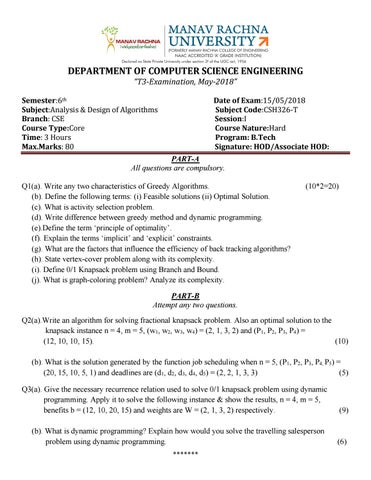DEPARTMENT OF COMPUTER SCIENCE ENGINEERING “T3-Examination, May-2018” Semester:6th Subject:Analysis & Design of Algorithms Branch: CSE Course Type:Core Time: 3 Hours Max.Marks: 80

Date of Exam:15/05/2018 Subject Code:CSH326-T Session:I Course Nature:Hard Program: B.Tech Signature: HOD/Associate HOD:

PART-A All questions are compulsory. Q1(a). Write any two characteristics of Greedy Algorithms. (b). Define the following terms: (i) Feasible solutions (ii) Optimal Solution. (c). What is activity selection problem. (d). Write difference between greedy method and dynamic programming. (e).Define the term ‘principle of optimality’. (f). Explain the terms ‘implicit’ and ‘explicit’ constraints. (g). What are the factors that influence the efficiency of back tracking algorithms? (h). State vertex-cover problem along with its complexity. (i). Define 0/1 Knapsack problem using Branch and Bound. (j). What is graph-coloring problem? Analyze its complexity.

(10*2=20)

PART-B Attempt any two questions. Q2(a).Write an algorithm for solving fractional knapsack problem. Also an optimal solution to the knapsack instance n = 4, m = 5, (w1, w2, w3, w4) = (2, 1, 3, 2) and (P1, P2, P3, P4) = (12, 10, 10, 15). (10) (b). What is the solution generated by the function job scheduling when n = 5, (P1, P2, P3, P4, P5) = (20, 15, 10, 5, 1) and deadlines are (d1, d2, d3, d4, d5) = (2, 2, 1, 3, 3) (5) Q3(a). Give the necessary recurrence relation used to solve 0/1 knapsack problem using dynamic programming. Apply it to solve the following instance & show the results, n = 4, m = 5, benefits b = (12, 10, 20, 15) and weights are W = (2, 1, 3, 2) respectively. (b). What is dynamic programming? Explain how would you solve the travelling salesperson problem using dynamic programming. *******

(9)

(6)

Q4(a). How is Prim’s algorithm different from Kruskal’s? Apply Prim’s algorithm to find the minimum spanning tree of the following graph: (7)

(b). Determine the cost & structure of an optimal binary search tree for set of n = 5 keys with the following possibilities: (8) i Pi qi

0 0.11

1 0.18 0.06

2 0.06 0.11

3 0.20 0.06

4 0.02 0.05

5 0.10 0.05

PART-C Attempt any two questions. Q5(a). Compare the iterative and recursive algorithm for back tracking method. Also give the control abstracts for both. (8) (b). Explain how the travelling salesperson problem can be solved using branch & bound method. (7) Q6(a). Write algorithm to place 8-queen’s in non-attacking position on 8 × 8 chess-board. Also draw its state space search tree. (10) (b). What is branch & bound method? Explain how isit different from backtracking. Q7(a). What is Hamilton cycle? How is it identified using backtracking method? (b). Solve the following vertex-cover problem for the given graph of set of edge as{(1,6), (1,2), (1,4), (2,3), (2,4), (6,7), (4,7), (7,8), (3,8), (3,5), (8,5)}

*******

(5) (7)

(8)